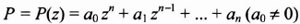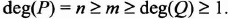## 10.3. Appendix: resultant of two polynomials

Let:two non-constant polynomials of variable z and with complex coefficients. We assume, without any loss of general information, that:We try to discover at what conditions the two polynomials P and Q admit a zero, or at least a non-constant factor, that they share. The results that we can establish are based on the lemma shown below. A demonstration of this lemma is found in [BEN 99].

LEMMA 10.1. – the polynomials P and Q have a zero in common if and only if there exist L and M non-constant polynomials in z that satisfy the following conditions:

LP + MQ = 0 and deg(M) < n and deg(L) < m.

By writing:and:the conditions of Lemma 10.1 is written: there are m + n complex numbers λ0,…,λm−1, μ0, …, μn−1 all not identically zero so that:In other words, the polynomial family:

is linearly dependent in the vectorial space of the complex polynomials ...

Get Digital Filters Design for Signal and Image Processing now with the O’Reilly learning platform.

O’Reilly members experience books, live events, courses curated by job role, and more from O’Reilly and nearly 200 top publishers.